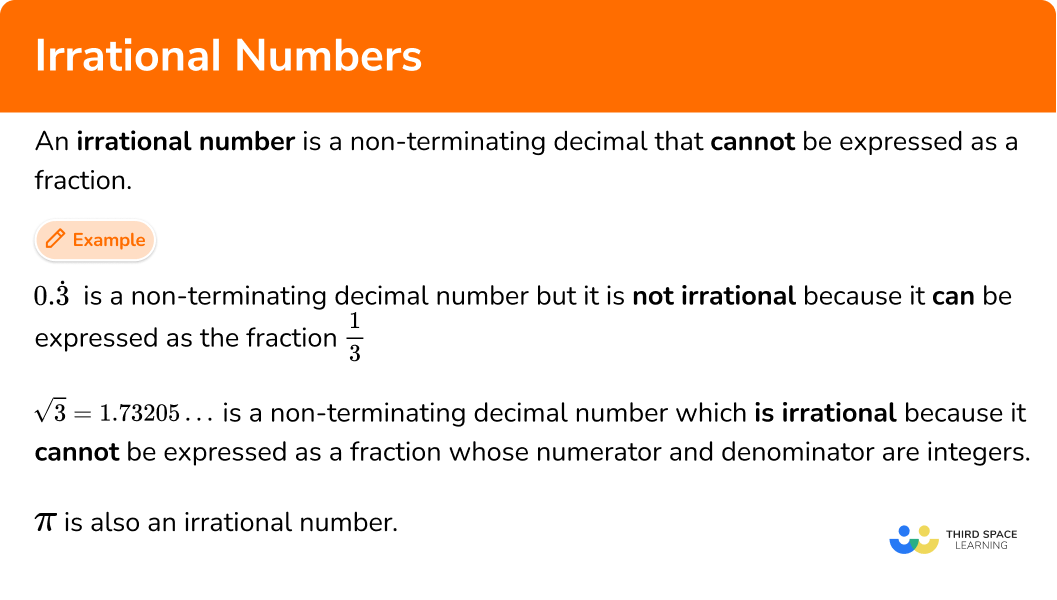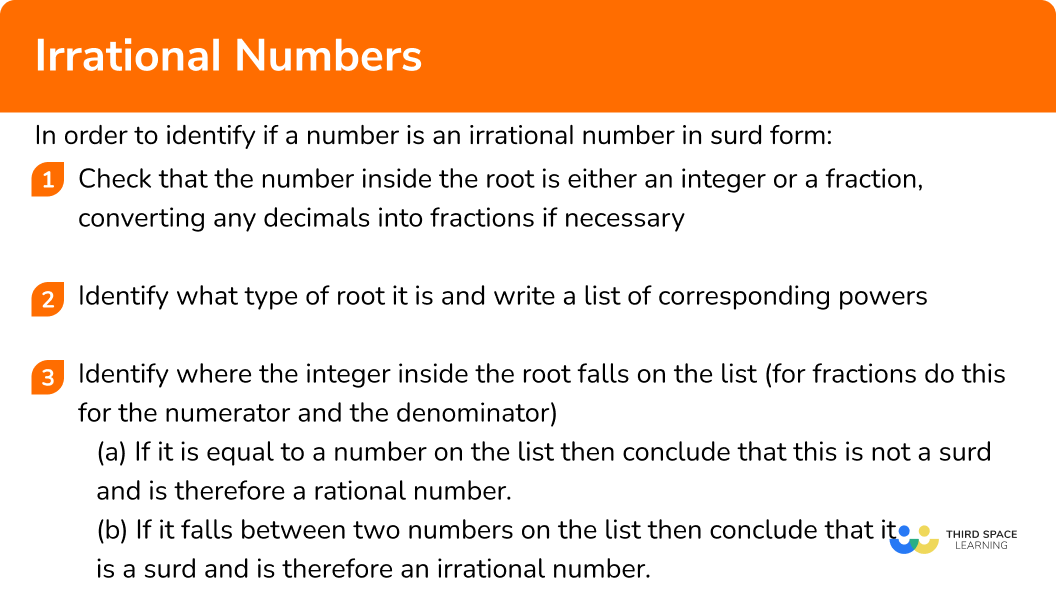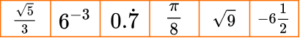GCSE Maths Number Types Of Numbers

Irrational Numbers

# Irrational Numbers

Here we will learn about irrational numbers, including the definition of an irrational number, examples of irrational numbers and how to identify irrational numbers.

There are also irrational numbers worksheets based on Edexcel, AQA and OCR exam questions, along with further guidance on where to go next if you’re still stuck.

## What is an irrational number?

An irrational number is a non-terminating decimal that cannot be expressed as a fraction. This is in opposition to the definition of a rational number which is any number that can be expressed as a fraction in the form \cfrac{a}{b} where a and b are integers (whole numbers) and b 0.

For example,

0.\dot{3} is a non-terminating decimal number which is not irrational because it can be expressed as the fraction \cfrac{1}{3}.

\sqrt{3}=1.73205… is a non-terminating decimal number which is irrational because it cannot be expressed as a fraction in the form \cfrac{a}{b} where a and b are integers.

There are several famous irrational numbers. These include,

Surds are types of irrational numbers. A surd is the root of a number which produces a non-terminating decimal.

For example,

\sqrt{9}=3 = rational

\sqrt{2}=1.414…= surd = irrational

\sqrt{0.25} = \sqrt{\cfrac{25}{100}} = \cfrac{\sqrt{25}}{\sqrt{100}} = \cfrac{5}{10} = \cfrac{1}{2} = rational

\sqrt{64}=4 = rational

\sqrt{30}=2.34034731932...= surd = irrational

### What is an irrational number?### Key points on irrational numbers

• Not all fractions are rational numbers

The definition of an irrational number is a number that cannot be expressed as a fraction in the form \cfrac{a}{b} where a and b are integers and b 0. But this does not mean that all fractions are rational.

For example, \cfrac{\sqrt{2}}{2} is a fraction which is also an irrational number because the numerator is irrational.

• The square root of a positive number that is not a square number is an irrational number

Square numbers (also known as perfect squares), are the numbers produced by the square of an integer.

1, 4, 9, 16, 25, 36…

When we take the square root of a square number the answer is rational; when we take the square root of any other positive integer the answer is irrational.

For example,

\sqrt{72}=8.48528... is an irrational number because 72 is NOT a square number.

\sqrt{12}= 3.46410... is an irrational number because 12 is NOT a square number. Note that \sqrt{12} can be simplified as \sqrt{4}\times\sqrt{3}=2\sqrt{3}, but as 3 is not a square number then 2\sqrt{3} produces an irrational number.

When we take the square root of the quotient of two square numbers the answer is rational.

For example, \sqrt{0.09}= \sqrt{\cfrac{9}{100}} = \cfrac{ \sqrt{9}}{ \sqrt{100}} = \cfrac{3}{10} = 0.3 .

• Other roots and surds

Similarly for other roots.

For example,

\sqrt{27}=3 , this cube root produces a rational number because 27 is a cube number.

\sqrt{50}=3.684031499… this cube root produces an irrational number because 50 is not a cube number.

\sqrt{0.008}= \sqrt{\cfrac{8}{1000}} = \cfrac{ \sqrt{8}}{ \sqrt{1000}} = \cfrac{2}{10} = 0.2 this cube root produces a rational number because 0.008 is the quotient of two cube numbers.

\sqrt{30}=2.34034731932… this 4th root produces an irrational number because 30 is not the result of an integer to the power of 4.

\sqrt{243}=3 , this 5th root produces a rational number because 243 is the result of an integer (3) to the power of 5.

• Recurring decimals

All irrational numbers are non-terminating decimals, but not all non-terminating decimals are irrational numbers.

Recurring decimals (also known as repeating decimals) are non terminating decimals (also known as non-repeating decimals) that are rational because they can be expressed as fractions in the form \frac{a}{b} where a and b are integers.

0.777... 0.\dot{7} is a non-terminating decimal number which is rational because it is a recurring decimal which can be expressed as the fraction \cfrac{7}{9}.

## How to identify if a number is an irrational number in surd form

In order to identify if a number is an irrational number in surd form:

1. Check that the number inside the root is either an integer or a fraction; converting any decimals into fractions if necessary.
2. Identify what type of root it is and write a list of corresponding powers.
3. Identify where the integer inside the root falls on the list (for fractions do this for the numerator and the denominator).
(a) If it is equal to a number on the list then conclude that this is not a surd and is therefore a rational number.
(b) If it falls between two numbers on the list then conclude that it is a surd and is therefore an irrational number.

### Explain how to identify if a number is an irrational number in surd form## Irrational numbers examples

### Example 1: identifying if the root of an integer is rational or irrational without a calculator

Is \sqrt{30} a rational or an irrational number?

1. Check that the number inside the root is either an integer or a fraction; converting any decimals into fractions if necessary.

30 is an integer.

2Identify what type of root it is and write a list of corresponding powers.

This is a square root.

The list of square numbers is 1,4,9,16,25,36,49…

3Identify where the integer inside the root falls on the list (for fractions do this for the numerator and the denominator).

(a) If it is equal to a number on the list then conclude that this is not a surd and is therefore a rational number.

(b) If it falls between two numbers on the list then conclude that it is a surd and is therefore an irrational number.

1,4,9,16,25 \; {\color{red}(30)} \; 36,49…

30 lies between two square numbers.

\sqrt{25}=5

\sqrt{36}=6

\sqrt{30} is an irrational number between 5 and 6.

### Example 2: identifying if the root of an integer is rational or irrational without a calculator

Is \sqrt{56} a rational or an irrational number?

56 is an integer.

This is a cube root.

The list of cube numbers, 1, 8, 27, 64, 125…

1, 8, 27 \; {\color{red}(56)} \; 64, 125…

56 lies between two cube numbers.

\sqrt{27}=3

\sqrt{64}=4

\sqrt{56} is an irrational number between 3 and 4.

### Example 3: identifying if the root of a fraction is rational or irrational without a calculator

Is \sqrt{\cfrac{36}{121}} a rational or an irrational number?

\cfrac{36}{121} is a fraction.

This is a square root.

The list of square numbers is 1,4,9,16,25,36,49,64,81,100,121,144…

1,4,9,16,25,{\color{red}36},49,64,81,100,{\color{red}121},144…

Both 36 and 121 are square numbers.

\sqrt{36}=6

\sqrt{121}=11

Therefore \sqrt{\cfrac{36}{121}}=\cfrac{\sqrt{36}}{\sqrt{121}}=\cfrac{6}{11} .

\sqrt{\cfrac{36}{121}} is a rational number.

### Example 4: identifying if the root of a decimal is rational or irrational without a calculator

Is \sqrt{2.5} a rational or an irrational number?

2.5 is a decimal so we must convert this to a fraction.

2.5=\cfrac{25}{10}

This is a square root.

The list of square numbers is 1,4,9,16,25,36,49…

1,4,9 \; {\color{red}(10)} \; 16, \; {\color{red}25}, 36, 49…

10 lies between two square numbers so \sqrt{10} is an irrational number.

25 is a square number so \sqrt{25}=5 which is a rational number.

However, both numbers need to be rational for the fraction to be rational.

As one of the numbers is irrational then the fraction will be irrational.

### Example 5: identifying if the root of a decimal is rational or irrational without a calculator

Is \sqrt{0.00005} a rational or an irrational number?

0.00005 is a decimal so we must convert this to a fraction.

0.00005=\cfrac{5}{100000}

This is a 5th root.

The list of integers to the power of 5,

1^{5}=1

2^{5}=32

...

10^{5}=100000

1, {\color{red}(5)}, 32, … \; {\color{red}100000}

5 lies between 1^{5} and 2^{5}, therefore \sqrt{5} is an irrational number.

100000 is 10^{5} therefore \sqrt{100000}=10 which is a rational number.

However, both numbers need to be rational for the fraction to be rational.

As one of the numbers is irrational then the fraction will be irrational.

### Example 6: estimating the value of a surd

Estimate the value of \sqrt{50} to one decimal place without using a calculator.

50 is an integer.

This is a square root.

The list of square numbers is 1,4,9,16,25,36,49,64,…

1,4,9,16,25,36,49,{\color{red}(50)},64,…

50 lies between two square numbers.

\sqrt{49}=7

\sqrt{64}=8

\sqrt{50} is an irrational number between 7 and 8.

As 50 is very close to 49 then \sqrt{50} will be very close to 7. Picturing a number line may also help you to estimate your answer.

Estimate \sqrt{50} \approx 7.1 .

(A calculator gives the answer 7.071067812… ).

### Example 7: estimating the value of a surd

Estimate the value of \sqrt{0.07} to one significant figure without using a calculator.

0.07 is a decimal so we must convert this to a fraction.

0.07=\cfrac{7}{100}

This is a square root.

The list of square numbers is 1,4,9,16…,81, 100.

1,4 \; {\color{red}(7)} \; 9,16…,81, {\color{red}100}

100 is a square number so \sqrt{100}=10 which is a rational number.

7 lies between two square numbers.

\sqrt{4}=2

\sqrt{9}=3

\sqrt{7} is an irrational number between 2 and 3.

7 is close to, but slightly above the middle of 4 and 9.

Therefore \sqrt{7} will be close to, but slightly above the middle of 2 and 3.

Estimate \sqrt{7} \approx 2.6 (in an exam answers from 2.5 to 2.8 would be accepted).

Estimate \sqrt{0.07} = \sqrt{\cfrac{7}{100}} = \cfrac{\sqrt{7}}{\sqrt{100}} \approx \cfrac{2.6}{10} = 0.26 = 0.3 \ (1sf) .

### Common misconceptions

• Assuming all fractions are rational numbers

All rational numbers can be written as fractions but not all fractions are rational numbers. If the fraction is in the form \cfrac{a}{b}, \ a and b are integers, and b 0 then the number is rational. However if a or b are not integers then the fraction could represent an irrational number.

For example, \cfrac{\sqrt{2}}{3} is a fraction which is irrational.

• Assuming all non-terminating decimals are irrational

All irrational numbers are non-terminating decimals. However not all non-terminating decimals are irrational numbers. Recurring decimals are non-terminating decimals which are rational.

For example,

\sqrt{5}=2.23606… is a non terminating decimal which is an irrational number.

\cfrac{8}{9}=0.888…=0.\dot{8} is a recurring decimal; a non terminating decimal which is a rational number.

• Writing a negative irrational number incorrectly

Here is an example of a positive irrational number, \sqrt{3}=1.73205…

To write the negative irrational number of the same magnitude you must write -\sqrt{3} and not \sqrt{-3} .

Try both of these on your calculator.

-\sqrt{3}=-1.73205…

\sqrt{-3}= you will get an error message on your calculator.

When you square a positive or a negative number, the answer is always positive.

For example,

5^{2}=25

(-5)^{2}=25

Therefore you cannot find the square root of a negative number. In maths we refer to the square root of a negative number as a number that is not real, or a number that is imaginary.

### Practice irrational numbers questions

1. Which of the following is irrational?

\sqrt{64}\sqrt{16}\sqrt{4}\sqrt{84}\sqrt{64}=8

\sqrt{16}=4

\sqrt{4}=2

\sqrt{84} = irrational (\sqrt{64}=8 and \sqrt{81}=9  so \sqrt{84} \;  lies between these).

2. Which of the following is rational?

\sqrt{44}\sqrt{144}44\pi\cfrac{4}{\sqrt{14}}\sqrt{144}=12 which is rational.

3. Which of the following is irrational?

\sqrt{64}\sqrt{64}\sqrt{64}None of these answers are irrational\sqrt{64}=8

\sqrt{64}=4

\sqrt{16}=2 and \sqrt{81}=3 so

\sqrt{64} is an irrational number between 2 and 3.

4. Which of the following is irrational?

\sqrt{36}\sqrt{0.36}\sqrt{3.6}None of these answers are irrational\sqrt{36}=6

\sqrt{0.36}=0.6

\sqrt{3.6}=\sqrt{\cfrac{36}{10}}=\cfrac{\sqrt{36}}{\sqrt{10}}=\cfrac{6}{\sqrt{10}}

As \sqrt{10} is irrational, this fraction is irrational.}

5. Which of the following is rational?

\sqrt{0.121}\sqrt{1.21}\sqrt{12.1}None of these answers are rational\sqrt{1.21}=\sqrt{\cfrac{121}{100}}=\cfrac{\sqrt{121}}{\sqrt{100}}=\cfrac{11}{10}= rational

6. Which of the following calculations gives an irrational answer?

\sqrt{21}\cfrac{8}{3}\sqrt{144}96 \div 7\cfrac{8}{3} = rational

\sqrt{144}=12 rational

96 \div 7 = \cfrac{96}{7} = rational

\sqrt{21} = irrational

\sqrt{16}=4 and \sqrt{25}=5, so \sqrt{21} = lies between these and is therefore irrational.

7. Which of the following is not irrational?

\cfrac{7}{2\pi}\sqrt{6}\cfrac{\sqrt{5}}{\sqrt{25}}\cfrac{3\pi}{2\pi}\cfrac{3\pi}{2\pi}=\cfrac{3}{2} = rational

Although \pi is an irrational number, the fraction can be simplified to cancel out \pi so this number is rational.

8. The value of the surd \sqrt{76} lies between which two integers?

7 and 88 and 96 and 738 and 391,4,9,16,25,36,49,64 \; {\color{red}(76)} \; 81,100,121,144,169

76 lies between 64 and 81 on the list of square numbers.

\sqrt{64}=8  and \sqrt{81}=9, so \sqrt{76} \; lies between 8 and 9.

9. Estimate the value of the irrational number \sqrt{23} correct to 1 decimal place.

4.25.14.84.51,4,9,16 \; {\color{red}(23)} \; 25,36,49,64,81,100,121,144,169

23 lies between 16 and 25 on the list of square numbers.

\sqrt{16}=4 and \sqrt{25}=5, so \sqrt{23} \; lies between 4 and 5.

23 is closer to 25 than it is to 16, so the answer will be closer to 5 than to 4.

10. State an irrational number that lies between 11 and 12.

4\pi\sqrt{145}11.5\sqrt{130}11^2=121, \ 12^2=144

Therefore the square root of any number between 121 and 144 will be an irrational number between 11 and 12.

\sqrt{130} is the correct answer from the multiple choice but there are many possible solutions. For example, \sqrt{125} or \sqrt{142.576}.

11. State an irrational number that lies between -5 and -3.

\sqrt{16}-\sqrt{10}\sqrt{-20}-4.\dot{3}(-3)^2=9, \ (-5)^2=25

Therefore the negative square root of any number between 9 and 25 will be an irrational number between -3 and -5, except the square number 16.

Remember \sqrt{-20} is an imaginary number; you will get an error message on your calculator.

### Irrational numbers GCSE questions

1. Below is a list of numbers.Circle the two irrational numbers in this list.

(1 mark)

\cfrac{\sqrt{5}}{3} and \cfrac{\pi}{8}

(1)

2. (a) The irrational number \sqrt{30} lies between which two integers?

(b) Estimate the answer to \sqrt{30} correct to one decimal place.

(3 marks)

(a)

\sqrt{25}=5 and \sqrt{36}=6

(1)

therefore \sqrt{30} = is an irrational number between 5 and 6.

(1)

(b)

5.5

(1)

3. (a) Express \sqrt{0.05} in the form \cfrac{\sqrt{a}}{b} where a and b are integers.

(b) Tyler says

0.05 is a rational number because it can be expressed as a fraction.”

Is Tyler correct?

(4 marks)

(a)

\sqrt{0.05} = \sqrt{\cfrac{5}{100}}

(1)

= \cfrac{\sqrt{5}}{\sqrt{100}} = \cfrac{\sqrt{5}}{10}

(1)

(b)

No

(1)

Correct justification

(1)

No. Tyer is wrong. All rational numbers can be expressed as fractions, but not all fractions are rational numbers.

No. Tyler is wrong because

\sqrt{4}=2 and \sqrt{9}=3 so \sqrt{5} = is an irrational number between 2 and 3.

Therefore \cfrac{\sqrt{5}}{10} is irrational.

No. Tyler is wrong.

Although \cfrac{\sqrt{5}}{10} is a fraction, it is irrational because \sqrt{5} is a surd.

## Learning checklist

You have now learned how to:

• Identify irrational numbers (with or without a calculator)

## Still stuck?

Prepare your KS4 students for maths GCSEs success with Third Space Learning. Weekly online one to one GCSE maths revision lessons delivered by expert maths tutors.

Find out more about our GCSE maths tuition programme.